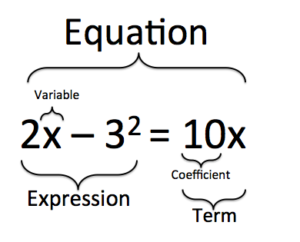## Translating and Solving Word Problems and Applications

### Learning Outcomes

• Translate word phrases to equations and solve
• Translate and solve applications

In previous chapters, we translated word phrases into equations. This skill will help you when you solve word problems. Previously, you translated phrases into expressions, and now we will translate phrases into mathematical equations so we can solve them.  But before we get started, let’s define some important terminology:

• variables:  Variables are symbols that stand for an unknown quantity, often represented with letters, like $x, y$, or $z$.
• coefficient: Sometimes a variable is multiplied by a number. This number is called the coefficient of the variable. For example, the coefficient of $3x$ is $3$.
• term: A single number, or variables and numbers connected by multiplication. For example, $-4, 6x$, and $x^2$ are all terms.
• expression: Groups of terms connected by addition and subtraction. For example, $2x^2-5$ is an expression.
• equation:  An equation is a mathematical statement that two expressions are equal. An equation will always contain an equal sign with an expression on each side. Think of an equal sign as meaning “the same as”. Some examples of equations are $y = mx +b$,  $\frac{3}{4}r = v^{3} - r$, and  $2(6-d) + f(3 +k) = \frac{1}{4}d$ .

The following figure shows how coefficients, variables, terms, and expressions all come together to make equations. In the equation $2x-3^2=10x$, the variable is $x$, a coefficient is $10$, a term is $10x$, and an expression is $2x-3^2$.Equation made of coefficients, variables, terms, and expressions.

## Translate Phrases into Equations

The first step in translating phrases into equations is to look for the word (or words) that translate(s) to the equal sign. The table below reminds us of some of the words that translate to the equal sign.

Equals (=)
is is equal to is the same as the result is gives was will be

Let’s review the steps we used to translate a sentence into an equation.

Translate a word sentence to an algebraic equation.

1. Locate the “equals” word(s). Translate to an equal sign.
2. Translate the words to the left of the “equals” word(s) into an algebraic expression.
3. Translate the words to the right of the “equals” word(s) into an algebraic expression.

In our first example, we will translate and solve a one-step equation.

### Example

Translate and solve: five more than $x$ is equal to $26$.

Solution:

 Translate. Five more than $x$   $\Rightarrow\quad{x+5}$is equal to   $\Rightarrow\quad{=}$ $26$   $\Rightarrow\quad{26}$ $x+5=26$ Subtract 5 from both sides. $x+5\color{red}{-5}=26\color{red}{-5}$ Simplify. $x=21$ Check:Is $26$ five more than $21$ ? $21+5\stackrel{\text{?}}{=}26$ $26=26\quad\checkmark$ The solution checks.

### Example

Translate and solve: The difference of $5p$ and $4p$ is $23$.

Watch this video for more examples of how to translate a phrase into an equation, then solve it.

## Translate and Solve Applications

In most of the application problems we solved earlier, we were able to find the quantity we were looking for by simplifying an algebraic expression. Now we will be using equations to solve application problems. We’ll start by restating the problem in just one sentence, then we’ll assign a variable, and then we’ll translate the sentence into an equation to solve. When assigning a variable, choose a letter that reminds you of what you are looking for.

### Example

The Robles family has two dogs, Buster and Chandler. Together, they weigh $71$ pounds.
Chandler weighs $28$ pounds. How much does Buster weigh?

### Devise a problem-solving strategy.

1. Read the problem. Make sure you understand all the words and ideas.
2. Identify what you are looking for.
3. Name what you are looking for. Choose a variable to represent that quantity.
4. Translate into an equation. It may be helpful to restate the problem in one sentence with all the important information. Then, translate the English sentence into an algebra equation.
5. Solve the equation using good algebra techniques.
6. Check the answer in the problem and make sure it makes sense.
7. Answer the question with a complete sentence.

Let’s take a look at the problem-solving strategy in action.

### example

Shayla paid $$24,575$ for her new car. This was$$875$ less than the sticker price. What was the sticker price of the car?

Now you can try translating an equation from a statement that represents subtraction.

In the following video, you will see another example of how to translate a phrase into an equation and solve.

## Contribute!

Did you have an idea for improving this content? We’d love your input.